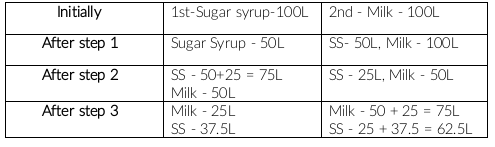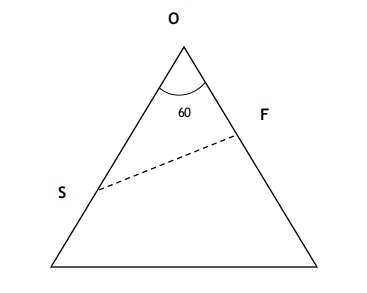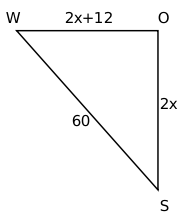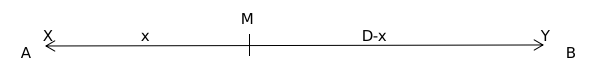0
956

# CAT Arithmetic Most Important Questions With Detailed Video Solutions PDF – Best Source For CAT 2023 Preparation

Arithmetic is one of the key topics in the CAT Quantitative Ability (QA) section. You can check out these top 20 Arithmetic questions from the CAT Previous year’s papers. Practising a good number of questions in CAT Arithmetic Questions will enhance your ability of solving the tough questions from Arithmetic. In this post, we will look into some important Arithmetic Questions for CAT QA. These are a good source for practice; If you want to practice these questions, you can download this Important CAT Arithmetic Questions PDF below, which is completely Free.

Question 1: A small jar contained water, lime and sugar in the ratio of 90:7:3. A glass contained only water and sugar in it. Contents of both (small jar and glass) were mixed in a bigger jar and the ratio of contents in the bigger jar was 85:5:10 (water, lime and sugar respectively). Find the percentage of water in the bigger jar?

a) 70

b) 75

c) 80

d) 72.5

e) 85

Solution:

The ratio of contents in the bigger jar was 85:5:10 (water, lime and sugar respectively).

Let volume of water be $85x$

Percentage of water in bigger jar = $\ \frac{\ 85x}{85x+5x+10x}\times100$ = $85$%

Option E is correct.

Question 2: Five students appeared for an examination. The average mark obtained by these five students is 40. The maximum mark of the examination is 100, and each of the five students scored more than 10 marks. However, none of them scored exactly 40 marks.
Based on the information given, which of the following MUST BE true?

a) At least, three of them scored a maximum of 40 marks

b) At least, three of them scored more than 40 marks

c) At least, one of them scored exactly 41 marks

d) At most, two of them scored more than 40 marks

e) At least, one of them scored less than 40 marks

Solution:

Option A) False, as it is mentioned no-one scored exactly 40 marks.

Option B) False, as only 1 student  or only 2 students can score above 40 and group average can be 40.

Case i:- 44, 39, 39, 39 & 39.

Case ii:- 42, 41, 39, 39 & 39.

Option C) False,  student’s scores could be 44, 39, 39, 39 & 39.

Option D) False, student’s scores could be 44, 43, 42, 32 & 39.

Option E) True, if scores of all students are more than 40. The average will be more than 40.

Question 3: In an examination, the average marks of students in sections A and B are 32 and 60, respectively. The number of students in section A is 10 less than that in section B. If the average marks of all the students across both the sections combined is an integer, then the difference between the maximum and minimum possible number of students in section A is

Solution:

Let the number of students in section A and B be a and b, respectively.

It is given, a = b – 10

$\ \ \frac{\ 32a+60b}{a+b}$ is an integer

$\ \ \frac{\ 32a+60\left(a+10\right)}{a+a+10}=k$

$\ \ \frac{\ 46a+300}{a+5}=k$

$k=\ \frac{\ 46\left(a+5\right)}{a+5}+\frac{70}{a+5}$

$k=\ \ 46+\frac{70}{a+5}$

a can take values 2, 5, 9, 30, 65

Difference = 65 – 2 = 63

Question 4: A glass contains 500 cc of milk and a cup contains 500 cc of water. From the glass, 150 cc of milk is transferred to the cup and mixed thoroughly. Next, 150 cc of this mixture is transferred from the cup to the glass. Now, the amount of water in the glass and the amount of milk in the cup are in the ratio

a) 1 : 1

b) 10 : 13

c) 3 : 10

d) 10 : 3

Solution:

Initially: a glass 500cc milk and a cup 500cc water

Step 1: 150 cc of milk is transferred to the cup from glass

After step 1: Glass – 350 cc milk, Cup – 150 cc milk and 500 cc water

Step 2: 150 cc of this mixture is transferred from the cup to the glass

After step 2:

Glass – 350 cc milk + 150 cc mixture with milk:water ratio 3:10

Cup – 500 cc mixture with milk:water ratio 3:10

water in glass : milk in cup = $\frac{10}{13}\times150\ :\ \frac{3}{13}\times500=1:1$

Question 5: Five students, including Amit, appear for an examination in which possible marks are integers between 0 and 50, both inclusive. The average marks for all the students is 38 and exactly three students got more than 32. If no two students got the same marks and Amit got the least marks among the five students, then the difference between the highest and lowest possible marks of Amit is

a) 22

b) 21

c) 24

d) 20

Solution:

The average marks for all the students is 38.

Sum = 5*38 = 190

To find the minimum marks scored by Amit, we need to maximise the score of remaining students.

Maximum scores sum of remaining students = 50 + 49 + 48 + 32 = 179

Minimum possible score of Amit = 190 – 179 = 11

It is given, Amit scored least. This implies maximum possible score of Amit is 31.

Difference = 31 – 11 = 20

Question 6: If a and b are non-negative real numbers such that a+ 2b = 6, then the average of the maximum and minimum possible values of (a+ b) is

a) 3

b) 4

c) 3.5

d) 4.5

Solution:

a + 2b = 6

From the above equation, we can say that maximum value b can take is 3 and minimum value b can take is 0.

a + b + b = 6

a + b = 6 – b

a + b is maximum when b is minimum, i.e. b = 0

Maximum value of a + b = 6 – 0 = 6

a + b is minimum when b is maximum, i.e. b = 3

Minimum value of a + b = 6 – 3 = 3

Average = $\ \frac{\ 6+3}{2}$ = 4.5

Question 7: There are two containers of the same volume, first container half-filled with sugar syrup and the second container half-filled with milk. Half the content of the first container is transferred to the second container, and then the half of this mixture is transferred back to the first container. Next, half the content of the first container is transferred back to the second container. Then the ratio of sugar syrup and milk in the second container is

a) 4 : 5

b) 6 : 5

c) 5 : 4

d) 5 : 6

Solution:Step 1: Half the content of the first container is transferred to the second container

Step 2: Half of the mixture of second container is transferred back to the first container

Step 3:  Half the content of the first container is transferred back to the second container

Sugar syrup : Milk in second container = 62.5 : 75 = 5 : 6

Question 8: In an election, there were four candidates and 80% of the registered voters casted their votes. One of the candidates received 30% of the casted votes while the other three candidates received the remaining casted votes in the proportion 1 : 2 : 3. If the winner of the election received 2512 votes more than the candidate with the second highest votes, then the number of registered voters was

a) 50240

b) 40192

c) 60288

d) 62800

Solution:

Let the number of registered votes be 100x

The number of votes casted = 80x

Votes received by one of the candidates = $\frac{30}{100}\times80x$ = 24x

Remaining votes = 80x – 24x = 56x

Votes received by other three candidates is $\frac{56x}{6},\frac{2\times56x}{6},\ \frac{\ 3\times56x}{6}$

It is given,

28x – 24x = 2512

4x = 2512

x = 628

The number of registered votes = 100x = 62800

Question 9: Manu earns ₹4000 per month and wants to save an average of ₹550 per month in a year. In the first nine months, his monthly expense was ₹3500, and he foresees that, tenth month onward, his monthly expense will increase to ₹3700. In order to meet his yearly savings target, his monthly earnings, in rupees, from the tenth month onward should be

a) 4400

b) 4200

c) 4300

d) 4350

Solution:

Savings target in a year = 550*12 = Rs 6600

Saving in first 9 months = 9(4000-3500) = Rs 4500

Saving for remaining 3 months should be 6600-4500, i.e. Rs 2100

Savings for each month in last 3 months = $\frac{2100}{3}$ = Rs 700

It is given, monthly expenses in last 3 months = Rs 3700

This implies, his monthly earnings from 10th month should be 3700+700, i.e. Rs 4400

Question 10: Pinky is standing in a queue at a ticket counter. Suppose the ratio of the number of persons standing ahead of Pinky to the number of persons standing behind her in the queue is 3 : 5. If the total number of persons in the queue is less than 300, then the maximum possible number of persons standing ahead of Pinky is

Solution:

Let the number of persons standing ahead and behind of Pinky be 3a and 5a.

Total number of persons = 3a + 5a + 1(including pinky) = 8a + 1

8a + 1 < 300

8a < 299

a < 37.375

Maximum value a can take is 37.

The maximum possible number of persons standing ahead of Pinky = 3a = 3*37 = 111

Question 11: Bob can finish a job in 40 days, if he works alone. Alex is twice as fast as Bob and thrice as fast as Cole in the same job. Suppose Alex and Bob work together on the first day, Bob and Cole work together on the second day, Cole and Alex work together on the third day, and then, they continue the work by repeating this three – day roster, with Alex and Bob working together on the fourth day, and so on. Then, the total number of days Alex would have worked when the job gets finished, is

Solution:

Let the efficiency of Bob be 3 units/day. So, Alex’s efficiency will be 6 units/day, and Cole’s will be 2 units/day.

Since Bob can finish the job in 40 days, the total work will be 40*3 = 120 units.

Since Alex and Bob work on the first day, the total work done = 3 + 6 = 9 units.

Similarly, for days 2 and 3, it will be 5 and 8 units, respectively.

Thus, in the first 3 days, the total work done = 9 + 5 + 8 = 22 units.

The work done in the first 15 days = 22*5 = 110 units.

Thus, the work will be finished on the 17th day(since 9 + 5 = 14 units are greater than the remaining work).

Since Alex works on two days of every 3 days, he will work for 10 days out of the first 15 days.

Then he will also work on the 16th day.

The total number of days = 11.

Question 12: Two ships are approaching a port along straight routes at constant speeds. Initially, the two ships and the port formed an equilateral triangle with sides of length 24 km. When the slower ship travelled 8 km, the triangle formed by the new positions of the two ships and the port became right-angled. When the faster ship reaches the port, the distance, in km, between the other ship and the port will be

a) 4

b) 12

c) 8

d) 6

Solution:Let S be the slower ship and F be the faster ship.

It is given that when S travelled 8 km, the positions of ships with the port is forming a right triangle.

Since one of the angles is 60(since one vertex is still part of the equilateral triangle),

the other two vertexes will have angles of 30 and 90.

The distance between O and S = 24 – 8 = 16

In triangle OFS, $\cos60^0\ =\ \frac{OF}{OS}$

Thus, OF = 8.

Thus in the time, S covered 8 km, F will cover 24 – 8 = 16 km.

Thus, the ratio of their speeds is 2:1,

Thus, when F covers 24 km, S will cover 12 km.

The correct option is B.

Question 13: Moody takes 30 seconds to finish riding an escalator if he walks on it at his normal speed in the same direction. He takes 20 seconds to finish riding the escalator if he walks at twice his normal speed in the same direction. If Moody decides to stand still on the escalator, then the time, in seconds, needed to finish riding the escalator is

Solution:

Let the speed of Moody be ‘x’ steps/sec and that of the escalator be ‘y’ steps/sec.

In 30 seconds, Moody will finish riding the escalator when going in the same direction.

Thus, total steps = 30(x+y)

If Moody’s speed becomes twice, the time becomes 20 seconds.

Thus, total steps = 20(2x+y)

Or    30x + 30y = 40x + 20y

Or    x = y

So, total steps = 60y.

Time taken by only escalator= 60y/y = 60s.

Question 14: Two cars travel from different locations at constant speeds. To meet each other after starting at the same time, they take 1.5 hours if they travel towards each other, but 10.5 hours if they travel in the same direction. If the speed of the slower car is 60 km/hr, then the distance traveled, in km, by the slower car when it meets the other car while traveling towards each other, is

a) 100

b) 90

c) 120

d) 150

Solution:

Both the cars take 1.5 hrs to meet when they travel towards each other.

It is given, speed of slower car is 60 km/hr

Therefore, distance covered by slower car before they meet = 60*1.5 = 90 km

Question 15: A group of N people worked on a project. They finished 35% of the project by working 7 hours a day for 10 days. Thereafter, 10 people left the group and the remaining people finished the rest of the project in 14 days by working 10 hours a day. Then the value of N is

a) 150

b) 23

c) 36

d) 140

Solution:

Let the unit of work done by 1 man in 1 hour and 1 day be 1 MDH unit (Man Day Hour).

Thus, in 7 hours per day for 10 days, the work done by N people =$N\times\ 7\times\ 10$ MDH units.

Since this is equal to 35% of the total work,

35% of the total work = $N\times\ 7\times\ 10$ MDH units.

Total work = $\frac{\left(N\times\ 100\times\ 7\times\ 10\right)}{35}=200\times N$ MDH units.

The work left = $200N-70N=130N$ MDH units.

Now, 10 people left the job. So, the number of people left = (N-10)

Since (N-10) people completed the rest of work in 14 days by working 10 hours a day,

$(N-10)\times\ 14\times\ 10=130N$

$10N=1400$

N = 140

Thus, the correct option is D.

Question 16: Working alone, the times taken by Anu, Tanu and Manu to complete any job are in the ratio 5 : 8 : 10. They accept a job which they can finish in 4 days if they all work together for 8 hours per day. However, Anu and Tanu work together for the first 6 days, working 6 hours 40 minutes per day. Then, the number of hours that Manu will take to complete the remaining job working alone is

Solution:

Let the time taken by Anu, Tanu and Manu be 5x, 8x and 10x hours.

Total work = LCM(5x, 8x, 10x) = 40x

Anu can complete 8 units in one hour

Tanu can complete 5 units in one hour

Manu can complete 4 units in one hour

It is given, three of them together can complete in 32 hours.

32(8 + 5 + 4) = 40x

x = $\frac{68}{5}$

It is given,

Anu and Tanu work together for the first 6 days, working 6 hours 40 minutes per day, i.e. 36 + 4 = 40 hours

40(8 + 5) + y(4) = 40x

4y = 24

y = 6

Manu alone will complete the remaining work in 6 hours.

Question 17: Two ships meet mid-ocean, and then, one ship goes south and the other ship goes west, both travelling at constant speeds. Two hours later, they are 60 km apart. If the speed of one of the ships is 6 km per hour more than the other one, then the speed, in km per hour, of the slower ship is

a) 20

b) 12

c) 18

d) 24

Solution:

Let the speeds of two ships be ‘x’ and ‘x+6’ km per hour

Distance covered in 2 hours will be 2x and 2x+12It is given,

$\left(2x\right)^2+\left(2x+12\right)^2=60^2$

$\left(x\right)^2+\left(x+6\right)^2=30^2$

$2x^2+12x+36=900$

$x^2+6x+18=450$

$x^2+6x-432=0$

Solving, we get x = 18

The speed of slower ship is 18 kmph

Question 18: Trains A and B start traveling at the same time towards each other with constant speeds from stations X and Y, respectively. Train A reaches station Y in 10 minutes while train B takes 9 minutes to reach station X after meeting train A. Then the total time taken, in minutes, by train B to travel from station Y to station X is

a) 6

b) 15

c) 10

d) 12

Solution:M – First meeting point

Let the speeds of trains A and B be ‘a’ and ‘b’, respectively.

$\frac{x}{a}=\ \frac{\ D-x}{b}$

It is given,

$\frac{D}{a}=10$ and $\frac{x}{b}=9$

$\frac{x}{\frac{D}{10}}=\ \frac{\ D-x}{\frac{x}{9}}$

$\frac{10x}{D}=\ \frac{\ 9D-9x}{x}$

$10x^2=\ \ 9D^2-9Dx$

$10x^2+9Dx-9D^2=\ 0$

Solving, we get $x=\frac{3D}{5}$

$\frac{x}{b}=9$

$\frac{3D}{b\times5}=9$

$\frac{D}{b}=15$

The total time taken by train B to travel from station Y to station X is 15 minutes.

Question 19: one pipe can fill a tank three times as fast as another pipe. If the two pipes together can fill the tank in 36 minutes, then the slower pipe will take how much time to fill the tank alone?

a) 81 minutes

b) 108 minutes

c) 192 minutes

d) 144 minutes

Solution:

Let slower pipe fill the tank at the rate x units/ minute.

Faster pipe will fill tank at the rate of 3x units/ minute

Number of units filled by both the pipes in a minute = 4x

Total time taken to fill tank = 36 minutes

capacity of tank = 36$\times\$4x = 144x

Time taken by slower pipe to fill the tank = $\frac{144x}{x}$ = 144 minutes

Question 20: With an average speed of 40 km/hr, a train reaches its destination in time. If it goes with an average speed of 30 km/hr, it is late by 36 minutes. The total distance travelled by train is :

a) 72 km

b) 78 km

c) 60 km

d) 40 km

Solution:

Let us consider with average speed of 40 km/hr, train reached its destination in time t hrs

with average speed of 30 km/hr, it takes t +$\frac{36}{60}$ hrs

Distance will be same in both the cases

40t = 30t + 18

10t = 18

t = $\frac{18}{10}$

Distance = 40t = 40$\times\ \frac{18}{10}$ = 72 km